# A right triangle can be isosceles

 Isosceles right triangle

What is an isosceles right triangle?

 ...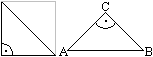... If you bisect a square by a diagonal, you get an isosceles right triangle. It therefore has a right angle and two sides of equal length.
Other names are 45-90-45 triangle or half square.

When a triangle is mentioned on this page, then the isosceles right triangle is meant.

Sizes of the triangle Top
The sizes are the hypotenuse AB, the legs AC and BC, the height h, the area A, the circumference U, the radius R of the circumference and the radius r of the inscribed circle.
If, for example, the hypotenuse a is given, the remaining quantities can be calculated.

 ...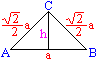... The legs are equal to sqr (2) / 2 * a, the height is h = a / 2.The height divides the triangle into again isosceles right-angled triangles.The area is A = a² / 4. The perimeter is U = [1 + sqr (2)] * a.

 ...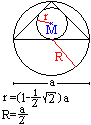... ...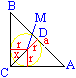... On the far left the circumference and the inscribed circle of the triangle are drawn.It is easy to see that the circumference has the radius R = a / 2.To calculate the incircle radius you have to go back a little:The relationships x = sqr (2) * r and CD = (1/2) a apply. Furthermore, CD = x + r.If you insert CD and x, you get (1/2) a = sqr (2) * r + r.The radius r is isolated, the denominator is made rational. The result is r = (1/2) [sqrt (2) -1] a.

Sequences of triangles Top

 ...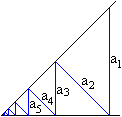... If you draw a zigzag line in the angular space of a 45 ° angle, you will get isosceles right triangles.If one gives the vertical distance with a1= a, then the "rungs" form the geometric sequence a1= a, a2= a / sqr (2), a3= a / [sqr (2)] 2, a4= a / [sqr (2)] ³, ....The corresponding sum, i.e. the geometric series, has the limit value [2 + sqr (2)] * a. That is roughly equal to 3.4 * a.

 ...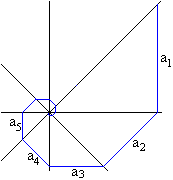... A vertical, a horizontal and the corresponding bisector form a straight line crossing of four straight lines.In this figure you can draw a line in the shape of a spiral.It is formed from the same lines as the zigzag line above.The length of the spiral approaches [2 + sqr (2)] * a as above.

Three isosceles right triangles form a triangular pyramid that is open at the bottom. The base is an equilateral triangle.
This pyramid appears in a cube, as the following stereo image shows. The picture shows two pyramids of this type.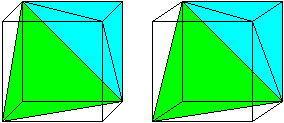If you fold the green pyramid up and place it on top of the blue pyramid (equilateral triangle on equilateral triangle), you get a double pyramid. This body is bounded by six isosceles right triangles.

Tangram puzzles Top
Classic tangram

 ...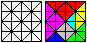... If you divide a square into 16 squares and draw the diagonals, you get 32 ​​isosceles right triangles. Certain triangles are combined to form the seven "tangram pieces". ...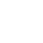...
The task is to place new figures from these "stones". You can find more on my Tangram page elsewhere.

Octagram
 ...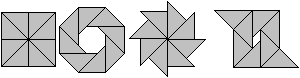... If you draw the diagonals and the center lines in a square, you get eight isosceles right-angled triangles. You can use them to create new figures.This puzzle is called the octagram (6).

7 triangle square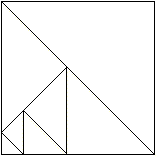Source: Ivan Skvarca, Journal of Recreational Mathematics 1998, sent by Wolfgang Schlueter

Color squares Top

 ...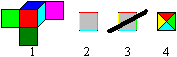... The set of colored dice from Mac Mahon is an attractive object for gadgets. These are the cubes that have six different colors in all combinations on the faces. A cube is shown on the left. If you want to go back from the cube to squares, you would consequently have to give the square sides four colors (2). This coloring is better extended to the isosceles right-angled triangles that lie against the sides (3, 4).

 ...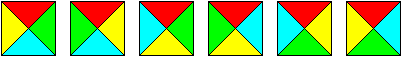... There are six different colored squares with four different colors.

 ...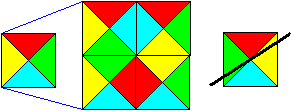... Mac Mahon's cube problem for squares is:Pick out a square and use four of the five remaining squares to make a square twice as large with the same colors on the outside. The same colors should meet on the inside.A solution is shown on the left. The mirror square to the first square remains.

These color squares are also called Wang tablets, because Hao Wang invented many variations of the tiling problem for them in 1961: the stones should be laid in such a way that the same colors always collide. More can be found in Book 4.

triangle ruler Top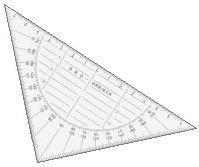The set square is a drawing device that did not come onto the market until the 1950s and has spread ever since. Today (2003) it is the Standard drawing device. Its spread reflects a development in school geometry. From my (imprecise) memory I will reproduce the development here, which is limited to high school.

At the beginning of the 1950s, a representative from Hanover traveled to elementary schools to advertise a new drawing device. He pointed out the following skills: One can comfortably >> cut lines in half, >> draw perpendiculars and parallels, >> draw angles. You saved other triangles and, in my opinion, also the compasses. However, the set square was initially quite expensive. He submitted a reference list of schools that had already introduced the triangle. Many vocational schools were among them.

The set square was actually superfluous for the grammar school, because in the classroom (following Euclidean geometry) it was only constructed, i.e. drawn with a compass and ruler. But the students quickly found out that the construction was cumbersome and drawing with the set square was faster. So they secretly used it when doing homework.
It was only when geometry lessons were increasingly prescribed later in grades 5 and 6 and the set triangle was present in every family that the purists among the teachers also gave in. The set square was tolerated as a drawing device in the grammar school and was introduced later. However, the teachers repeatedly emphasized that drawing with the set square was only a substitute for construction.
The construction as a geometric problem has become almost a marginal phenomenon in geometry lessons and is limited to the drawings that are not possible with the set square.

In retrospect, I see a similar development when the slide rule was replaced by the pocket calculator in the 1970s in the area of ​​number calculation.

A bit of nostalgia: a pair of compasses with a pencil stub (MADE IN ENGLAND, Pat. 1261866 & 1526566)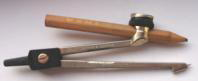who still knows him?

Polyabolos
With figures made up of several isosceles right-angled triangles, analogous to the pentominos or the polyiamonds, you can investigate many placement problems. They are called polyabolos. The tetrabolos made up of four triangles are the favorite, because the number 14 of the stones is neither too big nor too small. Further information can be found on the Polyabolos page elsewhere on my homepage.

Isosceles right-angled triangles also appear on other pages of my homepage, e.g. at Froebel Star or Heaven and Hell.

Isosceles right triangle on the internet Top

German

Education server South Tyrol
Measure angles with the set square

Wikipedia
Isosceles triangles, set square

English

Erich Friedman
Circles in tans

Eric W. Weisstein (MathWorld)
Isosceles Right Triangle, Polyabolo

Wikipedia Special right triangles # 45-45-90 Triangle, Polyabolo

credentials Top
(1) Martin Gardner: Mathematische Hexereien, Ullstein, Berlin / Frankfurt / Vienna, 1988 (ISBN 3 550065787)
(2) Bild der Wissenschaft 8/1979, (half-square multiples), page 102ff.
(3) Karl-Heinz Koch: ... lege games, DuMont, Cologne 1987 (ISBN 3-7701-2097-3)
(4) Friedrich L. Bauer: Invitation to Mathematics, Deutsches Museum, Munich 1999
(5) Bruno Kerst: Mathematical Games, Berlin 1933 (Reprint: Martin Sendet, Wiesbaden 1968)
(6) Ulrich Namisloh: Oktagram - graphic figure puzzle and placement game, Cologne 1984 (ISBN 3-7701-1636-4)

Feedback: Email address on my main page

URL of my homepage
http://www.mathematische-basteleien.de/